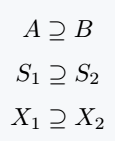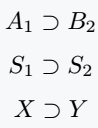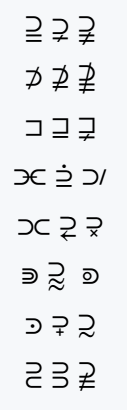# How to write superset(⊃, ⊇) in LaTeX?

Superset and Proper Superset are two different symbols. Many of you use Proper Superset as a superset set, which is the wrong approach.

Default \supseteq command is used to implement this symbol in the document.

Symbol Superset
Type of symbol Set Sumbol
Package No
Argument No
Latex command \supseteq
Example A \supseteq B → A ⊇ B
\documentclass{article}
\begin{document}
$A \supseteq B$
$S_1 \supseteq S_2$
$X_1 \supseteq X_2$
\end{document}

Output :## Proper Superset symbol in LaTeX

Proper superset symbol is to look like ⊃. For which there is a \supset command.

\documentclass{article}
\begin{document}
$A_1 \supset B_2$
$S_1 \supset S_2$
$X \supset Y$
\end{document}

Output :## Superset with different types of symbols in LaTeX

Different types of symbols are used with this symbol. Such as not equal, subset, equal, etc.

\documentclass{article}
\usepackage{stix}
\begin{document}
$\supseteqq \; \supsetneq \; \supsetneqq$
$\nsupset \; \nsupseteq \; \nsupseteqq$
$\sqsupset \; \sqsupseteq \; \sqsupsetneq$
$\supdsub \; \supedot \; \suphsol$
$\suphsub \; \suplarr \; \supmult$
$\Supset \; \supsetapprox \; \supsetcirc$
$\supsetdot \; \supsetplus \; \supsim$
$\supsub \; \supsup \; \varsupsetneqq$
\end{document}

Output :#### Md Jidan Mondal

LaTeX expert with over 10 years of experience in document preparation and typesetting. Specializes in creating professional documents, reports, and presentations using LaTeX.